﻿ M.Sc. Mathematics Coaching and Tuition Delhi University•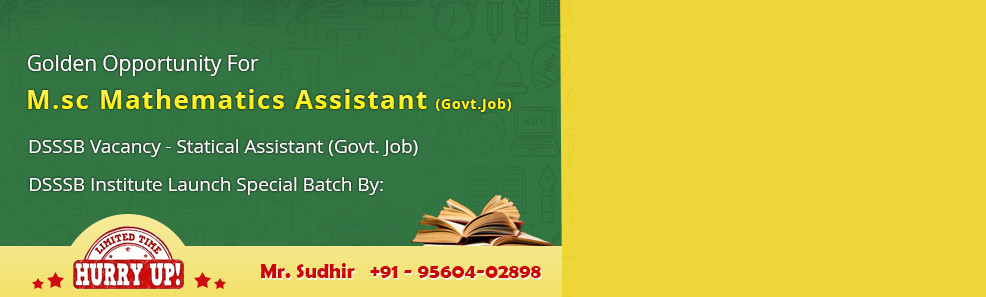•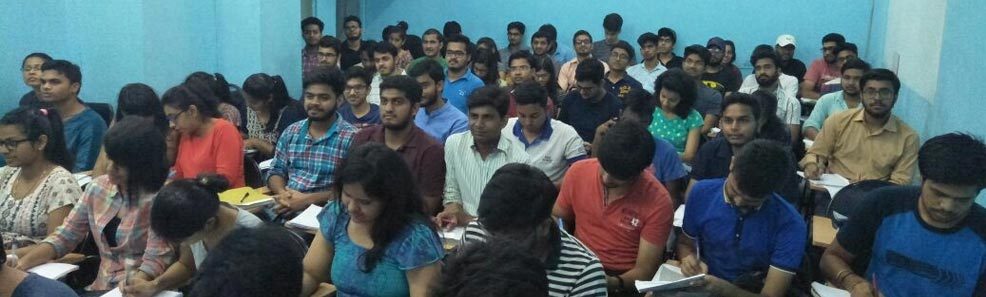•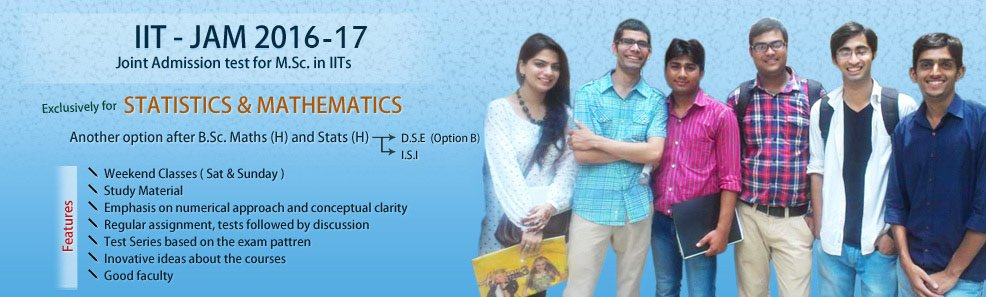•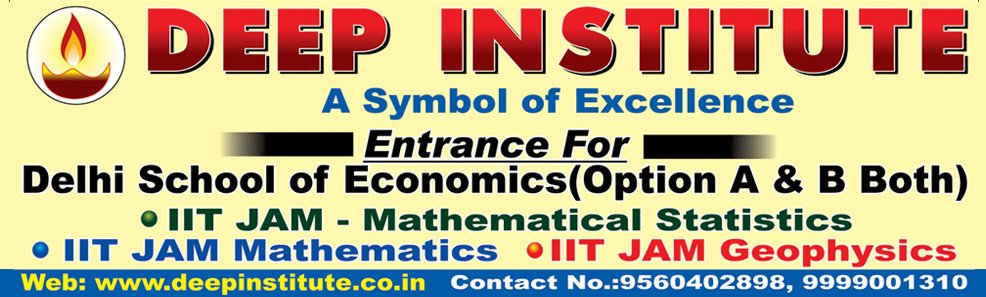•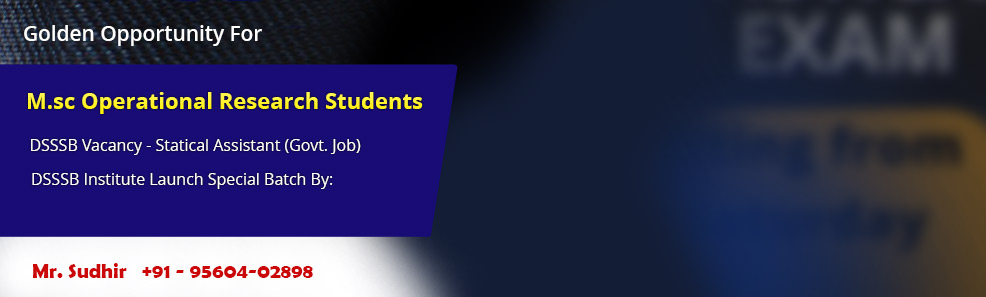•# MSc in Mathematics

Deep institute provides M.Sc. Maths coaching in Delhi for those pursuing the course from University of Delhi (DU). Candidates who holds a Master's degree (M.Sc.) in Mathematics can further specialize in any particular field or area of their interest. Today many Doctor of Philosophy and Master of Philosophy courses are available for Post Graduates (maths) Degree holders in the different areas of Mathematics. But to pursue any of these courses a candidate must meet the eligibility criteria specified for the particular course by the university offering the course.

A wide range of career opportunities are available in the field of research and teaching for those who successfully completes any one of these courses. Various multinational companies also offer lucrative jobs to these highly qualified candidates. Here are list of different doctoral level courses one can pursue after M.Sc. in Mathematics.

• Master of Philosophy in Applied Mathematics
• Master of Philosophy in Mathematical Science
• Master of Philosophy in Mathematics
• Master of Philosophy in Maths and Statistics
• Doctor of Philosophy in Applied Mathematics
• Doctor of Philosophy in Mathematics
• Doctor of Philosophy in Maths and Statistics

### Syllabus For Entrance Examination For M.A./M.Sc. Mathematics (Delhi University) :

Section - 1

• Elementary set theory, Finite, Countable and uncountable sets, Real number system as a complete ordered field, Archimedean property, Supremum, Infimum.
• Sequence and series, Convergence, Lim sup, Liminf.
• Bolzano weierstrass theorem, Heine Borel theorem.
• Continuity, Uniform continuity, Intermediate value theorem, Differentiability, Mean value theorem, Maclaurin's theorem and series, Taylor's series.
• Sequences and series of functions, Uniform convergence.
• Riemann sums and Riemann integral, Improper integrals.
• Monotonic functions, Types of discontinuity.
• Functions of several variables, Directional derivative, Partial derivative.
• Metric spaces, completeness, Total boundedness, Separability, Compactness, Connectedness.

Section - 2

• Eigenvalues and eigenvectors of matrices, Cayley-Hamilton theorem.
• Divisibility in Z, Congruences, Chinese remainder theorem, Euler's - function.
• Groups, Subgroups, Normal subgroups, Quotient groups, Homomorphisms, Cyclic groups, Permutation groups, Cayley's theorem, Class equations, Sylow theorems.
• Rings, Fields, Ideals prima and Maximal ideals, Quotient rings, Unique factorization domain, Polynomial rings and irreducibility criteria.
• Vector spaces, Subspaces, Linear dependence, Basis, Dimension, Algebra of linear transformations, Change of basis, Inner product spaces, Orthonormal Basis.

Section - 3

• Existence and uniqueness of solutions of initial value problems for first order ordinary differential equations, singular solutions of first order ordinary differential equations, System of first order ordinary differential equations, General theory of homogeneous and non-homogeneous linear ordinary differential equations, Variation of parameters, Sturm Liouville boundary value problem, green's function.
• Lagrange and Charpit methods for solving first order PDEs, Cauchy problem for first order PDEs, Classification of second order PDEs, General solution of higher order PDEs. With constant coefficients, Method of separation of variables for Laplace, Heat and wave equations.
• Numerical solutions of algebraic equations, method of iteration and newton Raphson method of convergence, solution of systems of linear algebraic equations of linear algebraic equations using Guass elimination and Guass-Seidel methods, finite differences, Lagrange, solutions of ODEs using Picard, Euler, Modified Euler and second order Runge-Kutta Methods.
• Velocity, acceleration, motion with constant and variables acceleration, Newton's Laws of Motion, simple Harmonic motion, motion of particle attached to elastic string, motion on inclined plane, motion of a projectile, angular velocity and acceleration, motion along a smooth vertical circle, work, energy and impulse, collision of elastic bodies, bodies falling in resisting medium, motion under action of central forces, central orbits, planetary motion, moment of inertia and couple, D' Alembart'sPrincipale.
• Equilibrium of particle and a system of particles, Mass centre and centres of gravity, Frictions, Equilibrium of rigid body, work and potential energy.

FOR PROPER GUIDANCE
Contact -- 09999001310 (SUDHIR SIR)

DEEP INSTITUTE
2513 Third Floor , Hudson Lane,
Opp - Laxmi Dairy ,
Near G.T.B. Nagar Metro Station Gate No – 4